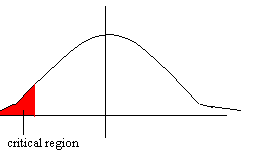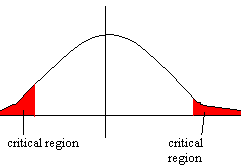## Title

One and Two Tailed Tests
Quick revise

Suppose we have a null hypothesis H0 and an alternative hypothesis H1. We consider the distribution given by the null hypothesis and perform a test to determine whether or not the null hypothesis should be rejected in favour of the alternative hypothesis.

There are two different types of tests that can be performed. A one-tailed test looks for an increase or decrease in the parameter whereas a two-tailed test looks for any change in the parameter (which can be any change- increase or decrease).

We can perform the test at any level (usually 1%, 5% or 10%). For example, performing the test at a 5% level means that there is a 5% chance of wrongly rejecting H0.

If we perform the test at the 5% level and decide to reject the null hypothesis, we say "there is significant evidence at the 5% level to suggest the hypothesis is false".

One-Tailed Test

We choose a critical region. In a one-tailed test, the critical region will have just one part (the red area below). If our sample value lies in this region, we reject the null hypothesis in favour of the alternative.

Suppose we are looking for a definite decrease. Then the critical region will be to the left. Note, however, that in the one-tailed test the value of the parameter can be as high as you like.Example

Suppose we are given that X has a Poisson distribution and we want to carry out a hypothesis test on the mean, l, based upon a sample observation of 3.

Suppose the hypotheses are:
H0: l = 9
H1: l < 9

We want to test if it is "reasonable" for the observed value of 3 to have come from a Poisson distribution with parameter 9. So what is the probability that a value as low as 3 has come from a Po(9)?

P(X < 3) = 0.0212 (this has come from a Poisson table)

The probability is less than 0.05, so there is less than a 5% chance that the value has come from a Poisson(3) distribution. We therefore reject the null hypothesis in favour of the alternative at the 5% level.

However, the probability is greater than 0.01, so we would not reject the null hypothesis in favour of the alternative at the 1% level.

Two-Tailed Test

In a two-tailed test, we are looking for either an increase or a decrease. So, for example, H0 might be that the mean is equal to 9 (as before). This time, however, H1 would be that the mean is not equal to 9. In this case, therefore, the critical region has two parts:Example

Lets test the parameter p of a Binomial distribution at the 10% level.

Suppose a coin is tossed 10 times and we get 7 heads. We want to test whether or not the coin is fair. If the coin is fair, p = 0.5 . Put this as the null hypothesis:

H0: p = 0.5
H1: p =(doesn' equal) 0.5

Now, because the test is 2-tailed, the critical region has two parts. Half of the critical region is to the right and half is to the left. So the critical region contains both the top 5% of the distribution and the bottom 5% of the distribution (since we are testing at the 10% level).

If H0 is true, X ~ Bin(10, 0.5).

If the null hypothesis is true, what is the probability that X is 7 or above?
P(X > 7) = 1 - P(X < 7) = 1 - P(X < 6) = 1 - 0.8281 = 0.1719

Is this in the critical region? No- because the probability that X is at least 7 is not less than 0.05 (5%), which is what we need it to be.

So there is not significant evidence at the 10% level to reject the null hypothesis.

Rate: